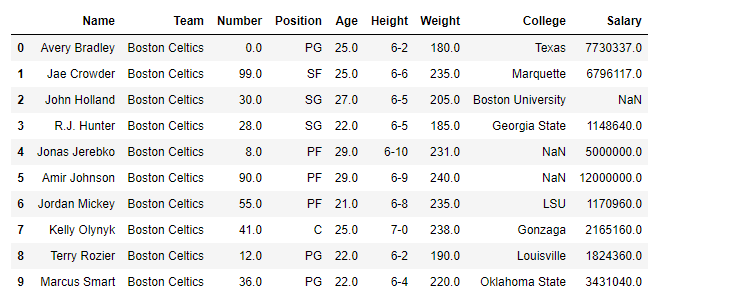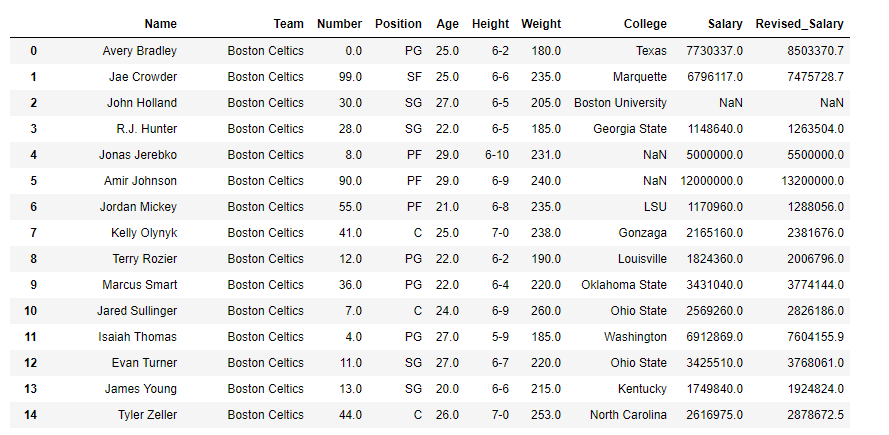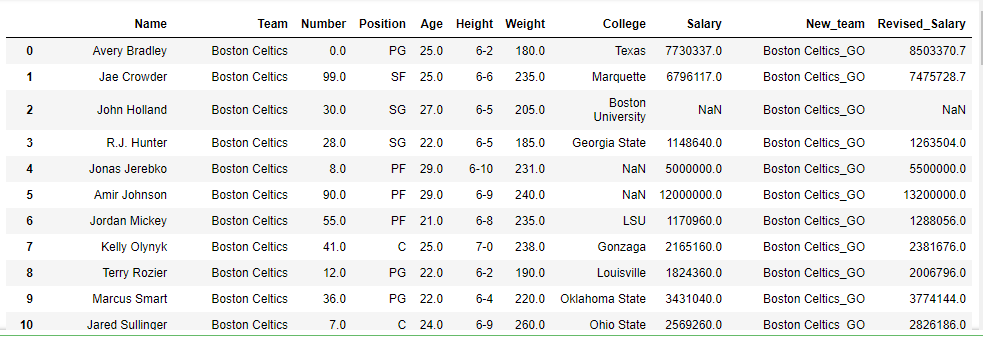Open In App

# Python | Pandas dataframe.assign()

Python is a great language for doing data analysis, primarily because of the fantastic ecosystem of data-centric python packages. Pandas is one of those packages and makes importing and analyzing data much easier.

`Dataframe.assign()` method assign new columns to a DataFrame, returning a new object (a copy) with the new columns added to the original ones. Existing columns that are re-assigned will be overwritten.

Length of newly assigned column must match the number of rows in the dataframe.

Syntax: DataFrame.assign(**kwargs)

Parameters:
kwargs : keywords are the column names. If the values are callable, they are computed on the DataFrame and assigned to the new columns. The callable must not change input DataFrame (though pandas don’t check it). If the values are not callable, (e.g. a Series, scalar, or array), they are simply assigned.

Returns: A new DataFrame with the new columns in addition to all the existing columns.

Example #1: Assign a new column called `Revised_Salary `with 10% increment of the original Salary.

 `# importing pandas as pd``import` `pandas as pd`` ` `# Making data frame from the csv file``df ``=` `pd.read_csv(``"nba.csv"``)`` ` `# Printing the first 10 rows of``# the data frame for visualization``df[:``10``]``# increase the salary by 10 % ``df.assign(Revised_Salary ``=` `lambda` `x: df[``'Salary'``]``                               ``+` `df[``'Salary'``]``/``10``)`

Output:Example #2: Assigning more than one column at a time

 `# importing pandas as pd``import` `pandas as pd`` ` `# Making data frame from the csv file``df ``=` `pd.read_csv(``"nba.csv"``)`` ` `# First column ='New_Team', this column``# will append '_GO' at the end of each team name.``# Second column ='Revised_Salary' will increase ``# the salary of all employees by 10 % ``df.assign(New_team ``=` `lambda` `x: df[``'Team'``]``+``'_GO'``, ``          ``Revised_Salary ``=` `lambda` `x: df[``'Salary'``] ``                             ``+` `df[``'Salary'``] ``/` `10``)`

Output: